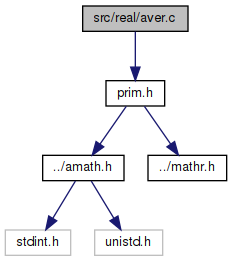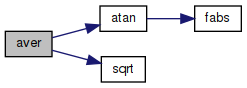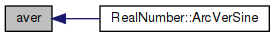amath  1.8.5 Simple command line calculator
aver.c File Reference

Inverse versed sine function. More...

`#include "prim.h"`
Include dependency graph for aver.c:Go to the source code of this file.

## Functions

double aver (double x)
Inverse versed sine function. More...

## Detailed Description

Inverse versed sine function.

Definition in file aver.c.

## ◆ aver()

 double aver ( double x )

Inverse versed sine function.

Definition at line 40 of file aver.c.

References atan(), and sqrt().

Referenced by RealNumber::ArcVerSine().

41 {
42  double a, b, c, d;
43
44  if (x < 0.0 || x > 2.0)
45  {
46  return NAN;
47  }
48
49  a = 2.0 * x - x * x;
50  b = sqrt(a);
51  c = 1.0 - x;
52
53  if (TRIG_INEXACT(c))
54  {
55  return INFP;
56  }
57
58  d = atan(b / c);
59  return d;
60 }
double atan(double x)
Inverse tangent function.
Definition: atan.c:103
#define INFP
Definition: mathr.h:51
#define TRIG_INEXACT(x)
Definition: prim.h:48
#define NAN
Definition: mathr.h:53
double sqrt(double x)
Square root function.
Definition: sqrt.c:119
Here is the call graph for this function:Here is the caller graph for this function: